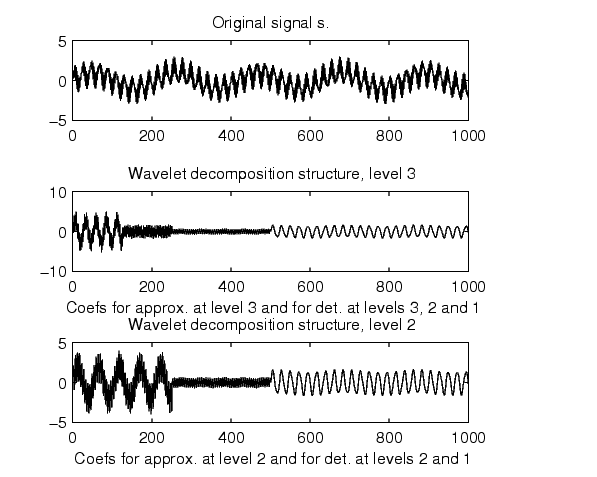Wavelet Toolboxupwlev

Single-level reconstruction of 1-D wavelet decomposition

Syntax

• [NC,NL,cA] = upwlev(C,L,'wname')
[NC,NL,cA] = upwlev(C,L,Lo_R,Hi_R)

Description

upwlev is a one-dimensional wavelet analysis function.

[NC,NL,cA] = upwlev(C,L,'wname') performs the single-level reconstruction of the wavelet decomposition structure [C,L] giving the new one [NC,NL], and extracts the last approximation coefficients vector cA.

[C,L] is a decomposition at level n = length(L)-2, so [NC,NL] is the same decomposition at level n-1 and cA is the approximation coefficients vector at level n.

'wname' is a string containing the wavelet name, C is the original wavelet decomposition vector, and L the corresponding bookkeeping vector (for detailed storage information, see wavedec).

Instead of giving the wavelet name, you can give the filters.

For [NC,NL,cA] = upwlev(C,L,Lo_R,Hi_R), Lo_R is the reconstruction low-pass filter and Hi_R is the reconstruction high-pass filter.

Examples

• % The current extension mode is zero-padding (see dwtmode).

% Perform decomposition at level 3 of s using db1.
[c,l] = wavedec(s,3,'db1');
subplot(311); plot(s);
title('Original signal s.');
subplot(312); plot(c);
title('Wavelet decomposition structure, level 3')
xlabel(['Coefs for approx. at level 3 ' ...
'and for det. at levels 3, 2 and 1'])

% One step reconstruction of the wavelet decomposition
% structure at level 3 [c,l], so the new structure [nc,nl]
% is the wavelet decomposition structure at level 2.
[nc,nl] = upwlev(c,l,'db1');
subplot(313); plot(nc);
title('Wavelet decomposition structure, level 2')
xlabel(['Coefs for approx. at level 2 ' ...
'and for det. at levels 2 and 1'])

% Editing some graphical properties,
% the following figure is generated.upcoef2 upwlev2